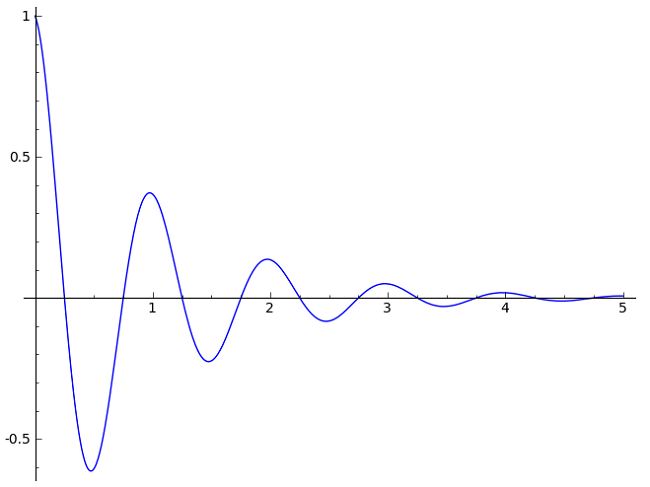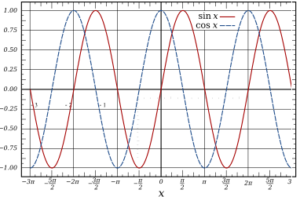# Damped Sine Wave: Definition, Example, FormulaA damped sine wave is a smooth, periodic oscillation with an amplitude that approaches zero as time goes to infinity. In other words, the wave gets flatter as the x-values get larger.

Watch the video for an overview, the formula, and a demonstration of what happens when you change the damping factor:

Damped sine waves are often used to model engineering situations where a harmonic oscillator is losing energy with each oscillation. For example: a bouncing tennis ball or a swinging clock pendulum.

The term damped sine wave refers to both damped sine and damped cosine waves, or a function that includes a combination of sine and cosine waves. A cosine curve (blue in the image below) has exactly the same shape as a sine curve (red), only shifted half a period. Where a sine wave crosses the y-axis at y = 0, the cosine wave crosses it at y = 1.Notice though, that the sine and cosine waves in the above image are not damped: they are a uniform height as they move from left to right.

## Formula for a Damped Sine Wave

A sine wave may be damped in any of an infinite number of ways, but the most common form is exponential damping. If your sine curve is exponentially damped, drawing a line from peak to peak will result in an exponential decay curve, which has the general formula N(t) = A e(kt). Draw a curve from peak to peak, and you’ll see the exponential function.Exponential damping y(t) = e-t · cos (2 π t), with the exponential decay curve shown in red.

We can write a general equation for an exponentially damped sinusoid asIn some cases the equation can be simplified to:Where:

• A is the initial amplitude (the highest peak),
• λ is the decay constant,
• Φ is the phase angle (at t = 0)
• ω is the angular frequency.

Notice that the curve in the above example crosses the y-axis at x = 1 (since A = 1 in this particular example) and that the amplitude (height) goes to zero as x goes to infinity.

## References

Guido, Mueller. Damped Simple Harmonic Motion. Retrieved from http://www.phys.ufl.edu/~mueller/PHY2048/2048_Chapter16_F08_wHitt_Part1.pdf on April 18, 2019.
Townsend, Lee. Analyzing Damped Oscillations. Retrieved from http://uhaweb.hartford.edu/ltownsend/Analyzing_Graphs_of_Damped_Oscillation_Data.pdf on April 18, 2019.

CITE THIS AS:
Stephanie Glen. "Damped Sine Wave: Definition, Example, Formula" From StatisticsHowTo.com: Elementary Statistics for the rest of us! https://www.statisticshowto.com/calculus-definitions/damped-sine-wave/